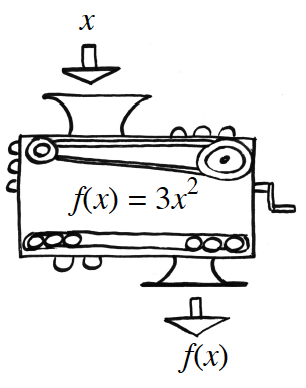### Home > PC3 > Chapter 1 > Lesson 1.1.6 > Problem1-60

1-60.A function machine is shown at right.

1. If the input is $x=-2$, what is the output?
If the input is $x=10$, what is the output?

If $f(x)=3x^2$, what is $f(−2)$? $f(10)$?

2. If $27$ is the output, what was the input?
If $147\$is the output, what was the input?

The output is $f(x)$, so solve $f(x)=27$, which is equivalent to $3x^2=27$.

3. If the equation for determining the output from a given input is $f(x)=3x^2$, what is the equation for determining the input from a given output?

If the output is a value, $y$, then solve the equation $y=3x^2$ for $x$.